Скачать презентацию Equilibrium Non reversible reactions Some chemical

d25bd2e8108465f577d3dc4255c8d168.ppt

• Количество слайдов: 44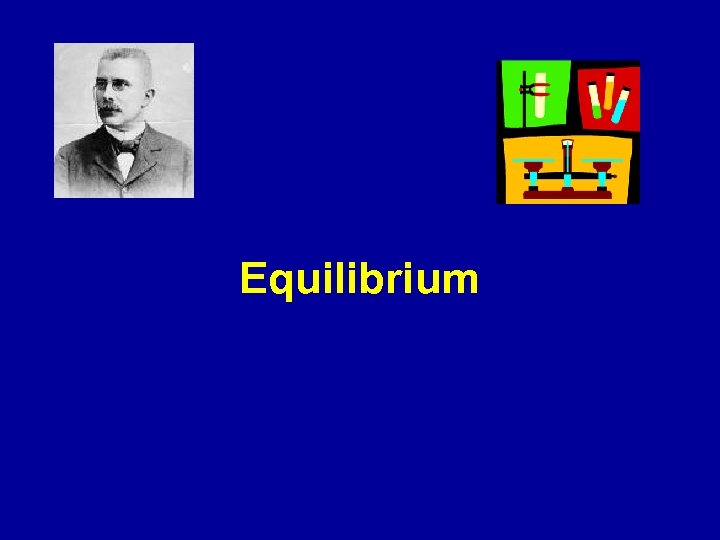Equilibrium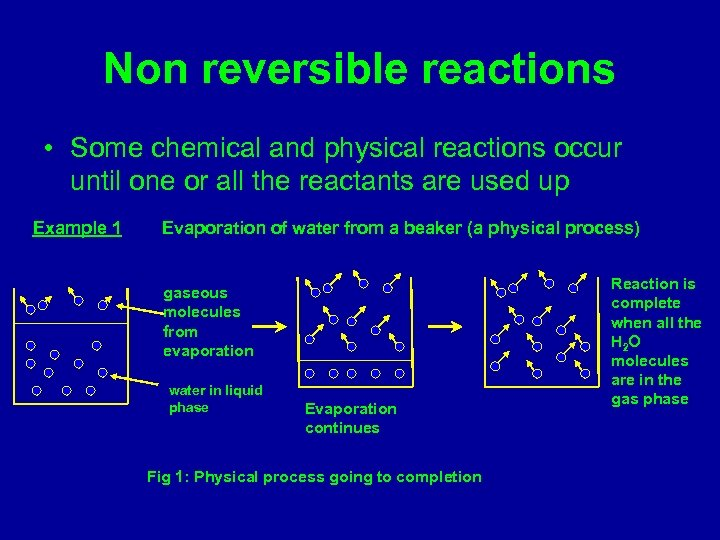Non reversible reactions • Some chemical and physical reactions occur until one or all the reactants are used up Example 1 Evaporation of water from a beaker (a physical process) gaseous molecules from evaporation water in liquid phase Evaporation continues Fig 1: Physical process going to completion Reaction is complete when all the H 2 O molecules are in the gas phase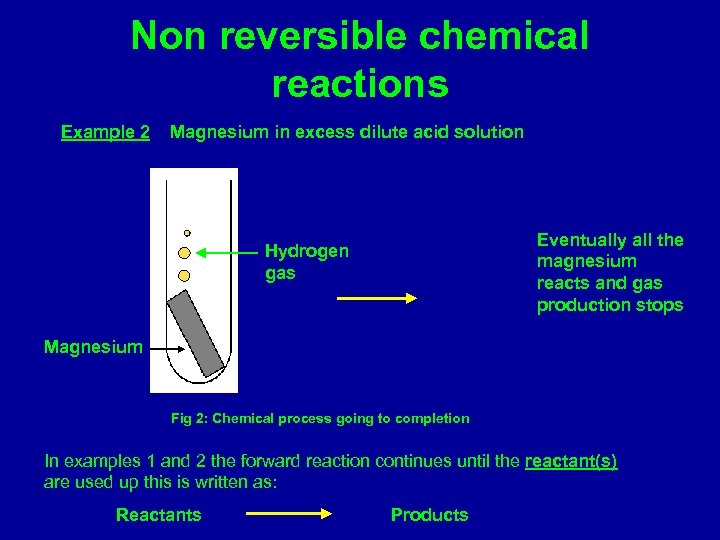Non reversible chemical reactions Example 2 Magnesium in excess dilute acid solution Eventually all the magnesium reacts and gas production stops Hydrogen gas Magnesium Fig 2: Chemical process going to completion In examples 1 and 2 the forward reaction continues until the reactant(s) are used up this is written as: Reactants Products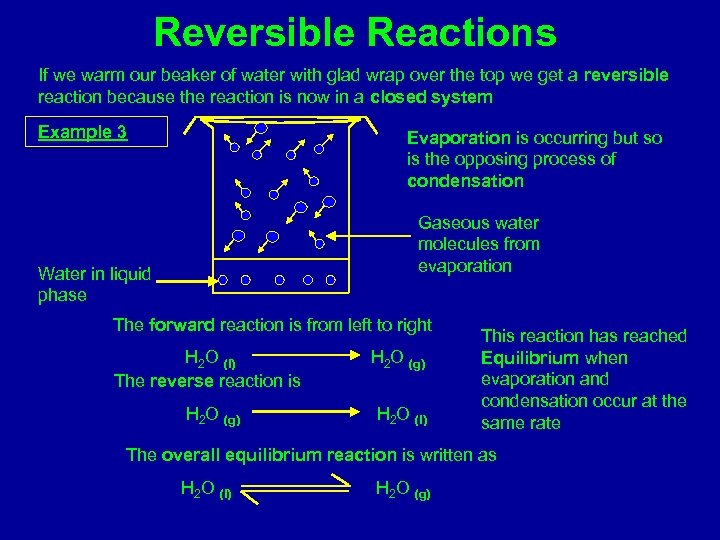Reversible Reactions If we warm our beaker of water with glad wrap over the top we get a reversible reaction because the reaction is now in a closed system Example 3 Evaporation is occurring but so is the opposing process of condensation Gaseous water molecules from evaporation Water in liquid phase The forward reaction is from left to right H 2 O (l) The reverse reaction is H 2 O (g) H 2 O (l) This reaction has reached Equilibrium when evaporation and condensation occur at the same rate The overall equilibrium reaction is written as H 2 O (l) H 2 O (g)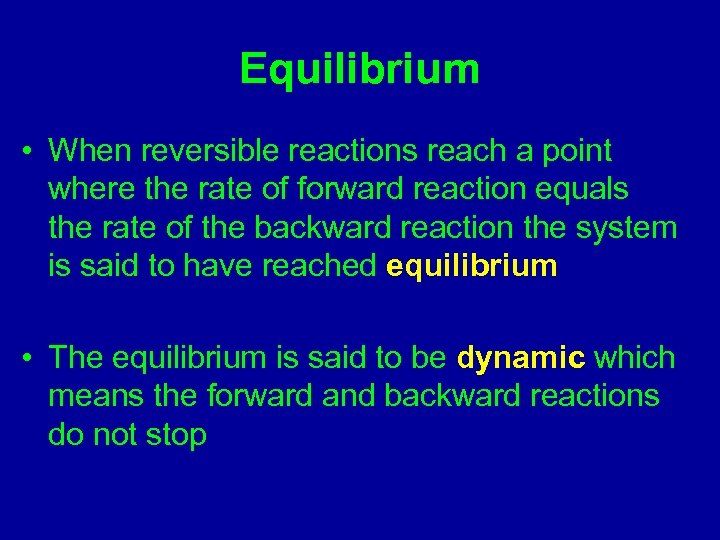Equilibrium • When reversible reactions reach a point where the rate of forward reaction equals the rate of the backward reaction the system is said to have reached equilibrium • The equilibrium is said to be dynamic which means the forward and backward reactions do not stop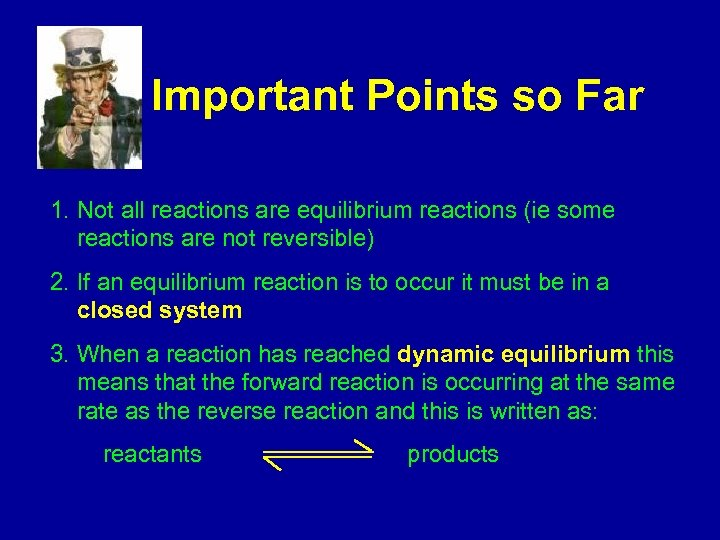Important Points so Far 1. Not all reactions are equilibrium reactions (ie some reactions are not reversible) 2. If an equilibrium reaction is to occur it must be in a closed system 3. When a reaction has reached dynamic equilibrium this means that the forward reaction is occurring at the same rate as the reverse reaction and this is written as: reactants products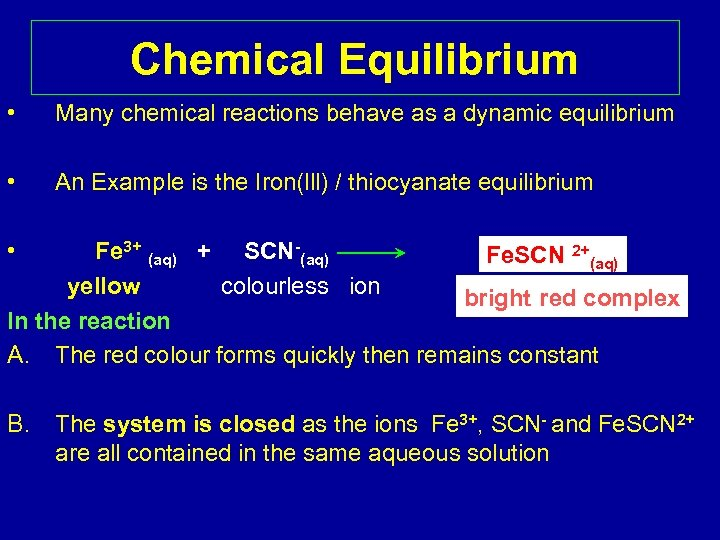Chemical Equilibrium • Many chemical reactions behave as a dynamic equilibrium • An Example is the Iron(lll) / thiocyanate equilibrium • Fe 3+ (aq) + SCN-(aq) Fe. SCN 2+(aq) yellow colourless ion bright red complex In the reaction A. The red colour forms quickly then remains constant B. The system is closed as the ions Fe 3+, SCN- and Fe. SCN 2+ are all contained in the same aqueous solution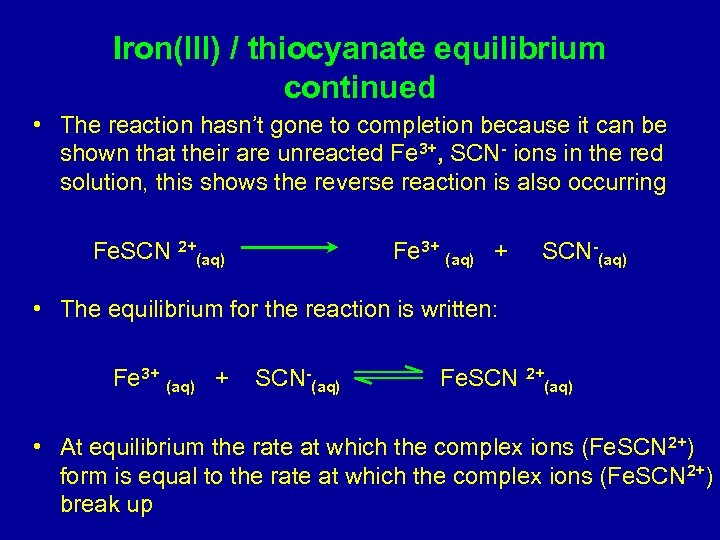Iron(lll) / thiocyanate equilibrium continued • The reaction hasn’t gone to completion because it can be shown that their are unreacted Fe 3+, SCN- ions in the red solution, this shows the reverse reaction is also occurring Fe. SCN 2+(aq) Fe 3+ (aq) + SCN-(aq) • The equilibrium for the reaction is written: Fe 3+ (aq) + SCN-(aq) Fe. SCN 2+(aq) • At equilibrium the rate at which the complex ions (Fe. SCN 2+) form is equal to the rate at which the complex ions (Fe. SCN 2+) break up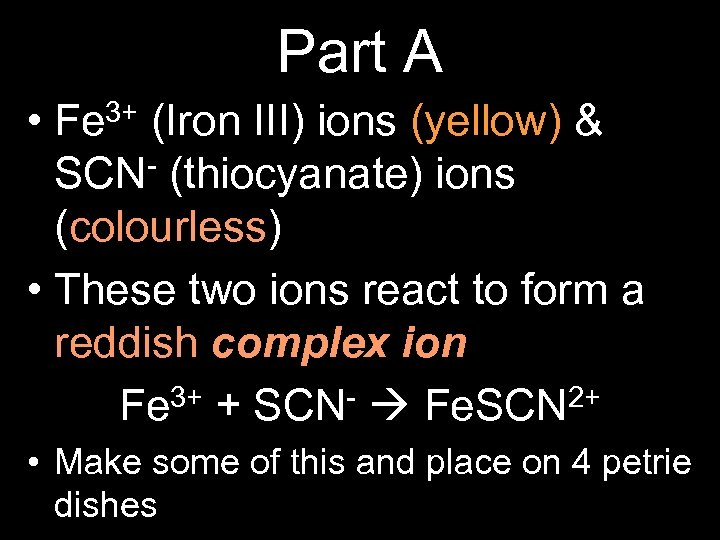Part A • Fe 3+ (Iron III) ions (yellow) & SCN- (thiocyanate) ions (colourless) • These two ions react to form a reddish complex ion: 3+ + SCN- Fe. SCN 2+ Fe • Make some of this and place on 4 petrie dishes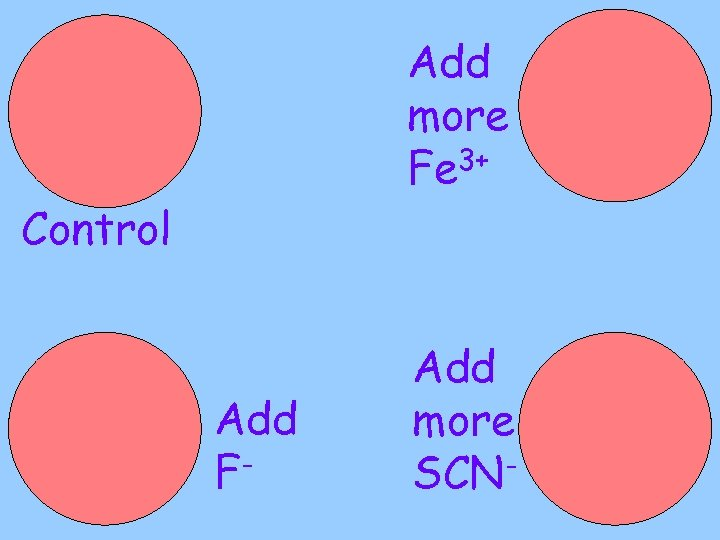Add more Fe 3+ Control Add F- Add more SCN-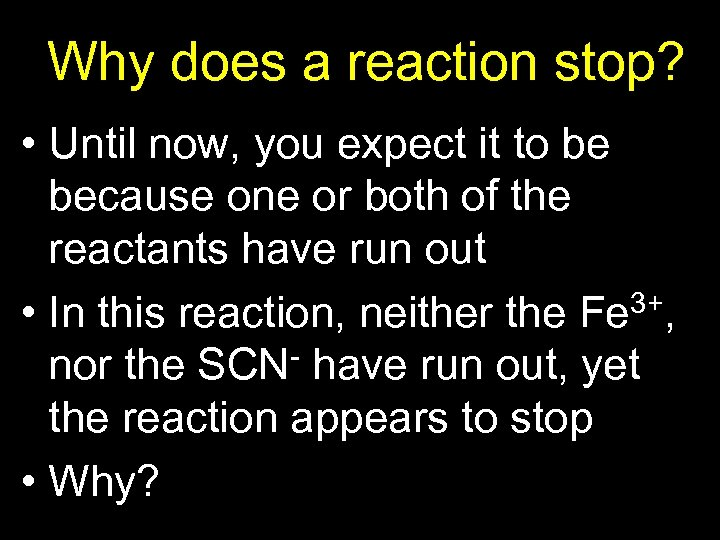Why does a reaction stop? • Until now, you expect it to be because one or both of the reactants have run out • In this reaction, neither the Fe 3+, nor the SCN- have run out, yet the reaction appears to stop • Why?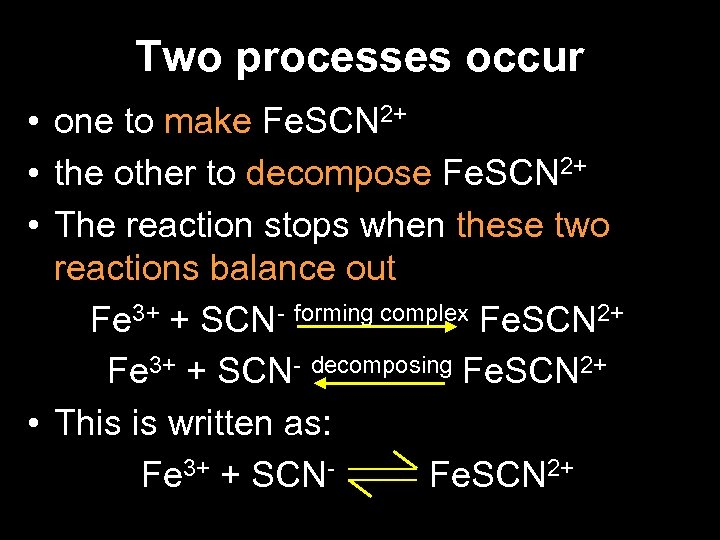Two processes occur • one to make Fe. SCN 2+ • the other to decompose Fe. SCN 2+ • The reaction stops when these two reactions balance out Fe 3+ + SCN- forming complex Fe. SCN 2+ Fe 3+ + SCN- decomposing Fe. SCN 2+ • This is written as: Fe 3+ + SCNFe. SCN 2+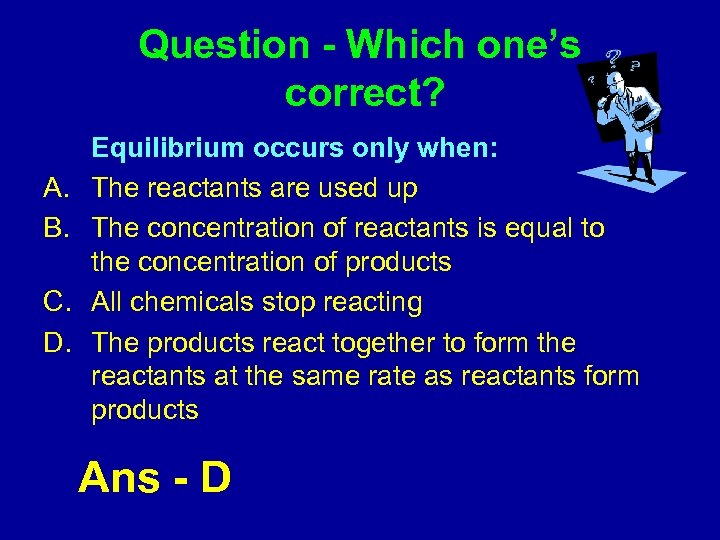Question - Which one’s correct? A. B. C. D. Equilibrium occurs only when: The reactants are used up The concentration of reactants is equal to the concentration of products All chemicals stop reacting The products react together to form the reactants at the same rate as reactants form products Ans - D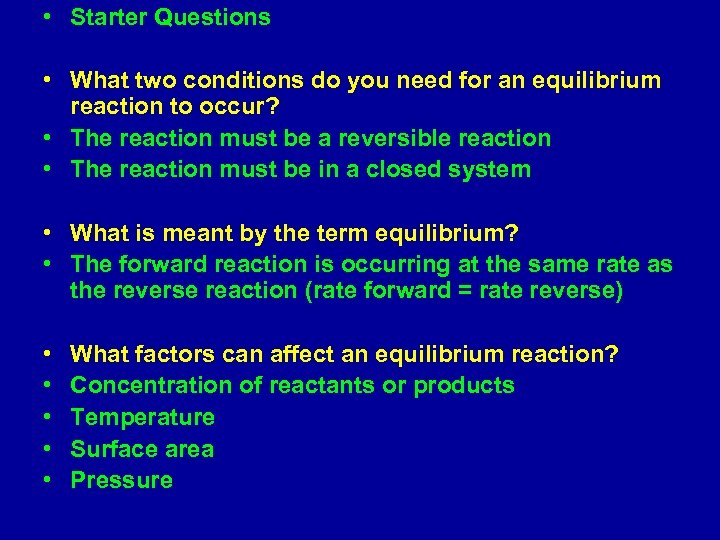• Starter Questions • What two conditions do you need for an equilibrium reaction to occur? • The reaction must be a reversible reaction • The reaction must be in a closed system • What is meant by the term equilibrium? • The forward reaction is occurring at the same rate as the reverse reaction (rate forward = rate reverse) • • • What factors can affect an equilibrium reaction? Concentration of reactants or products Temperature Surface area Pressure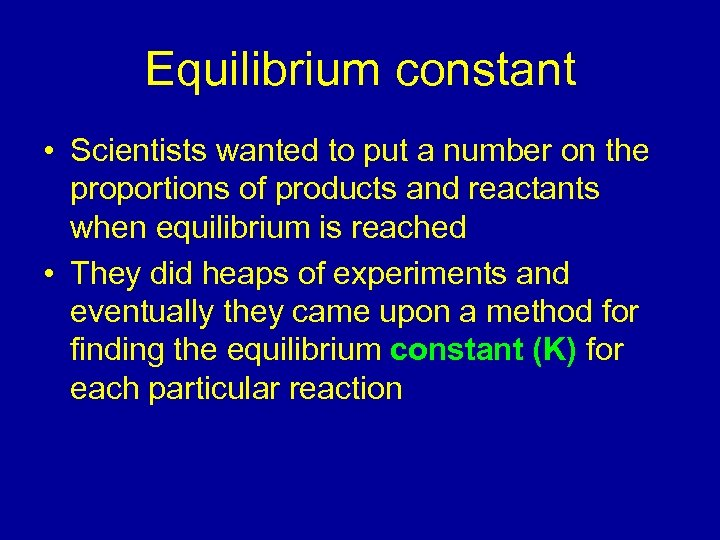Equilibrium constant • Scientists wanted to put a number on the proportions of products and reactants when equilibrium is reached • They did heaps of experiments and eventually they came upon a method for finding the equilibrium constant (K) for each particular reaction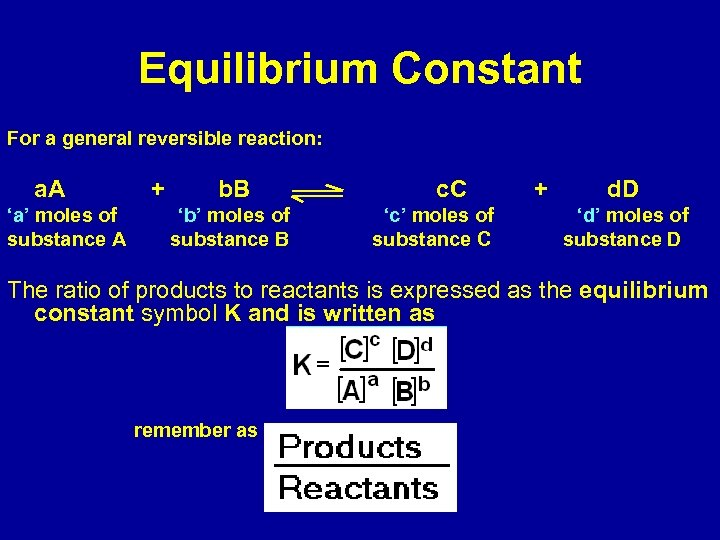Equilibrium Constant For a general reversible reaction: a. A ‘a’ moles of substance A + b. B ‘b’ moles of substance B c. C ‘c’ moles of substance C + d. D ‘d’ moles of substance D The ratio of products to reactants is expressed as the equilibrium constant symbol K and is written as remember as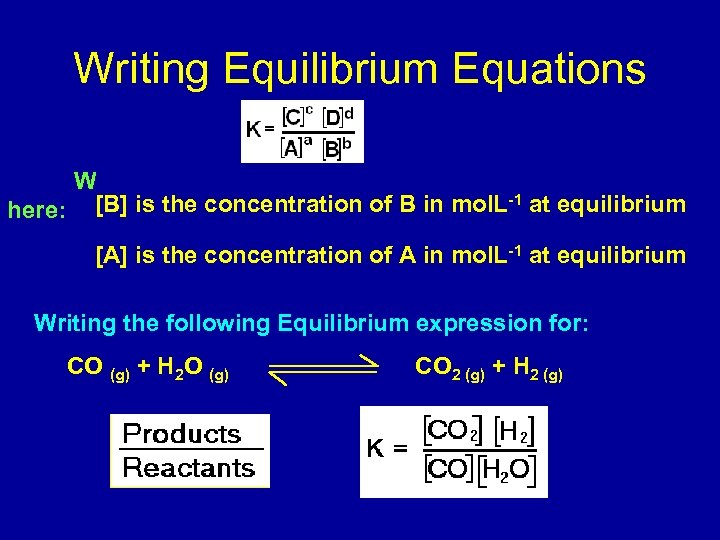Writing Equilibrium Equations W -1 here: [B] is the concentration of B in mol. L at equilibrium [A] is the concentration of A in mol. L-1 at equilibrium Writing the following Equilibrium expression for: CO (g) + H 2 O (g) CO 2 (g) + H 2 (g)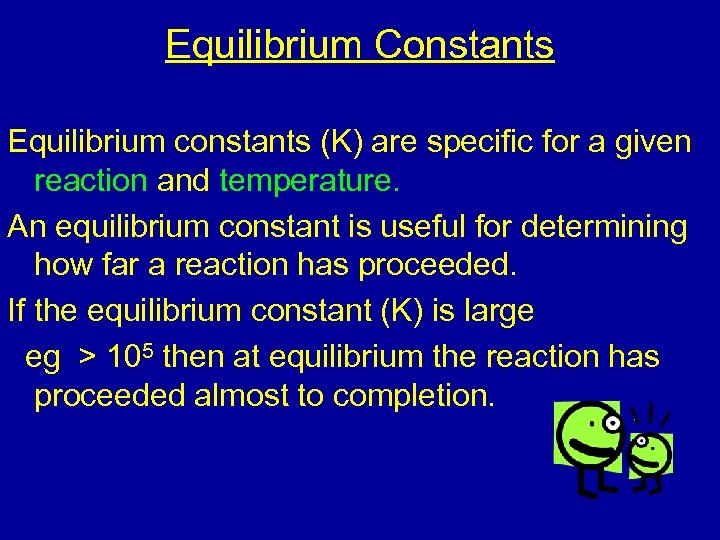Equilibrium Constants Equilibrium constants (K) are specific for a given reaction and temperature. An equilibrium constant is useful for determining how far a reaction has proceeded. If the equilibrium constant (K) is large eg > 105 then at equilibrium the reaction has proceeded almost to completion.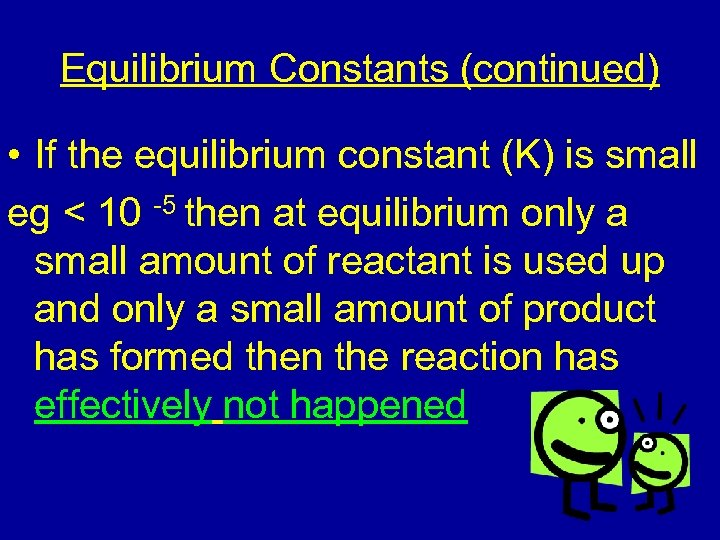Equilibrium Constants (continued) • If the equilibrium constant (K) is small eg < 10 -5 then at equilibrium only a small amount of reactant is used up and only a small amount of product has formed then the reaction has effectively not happened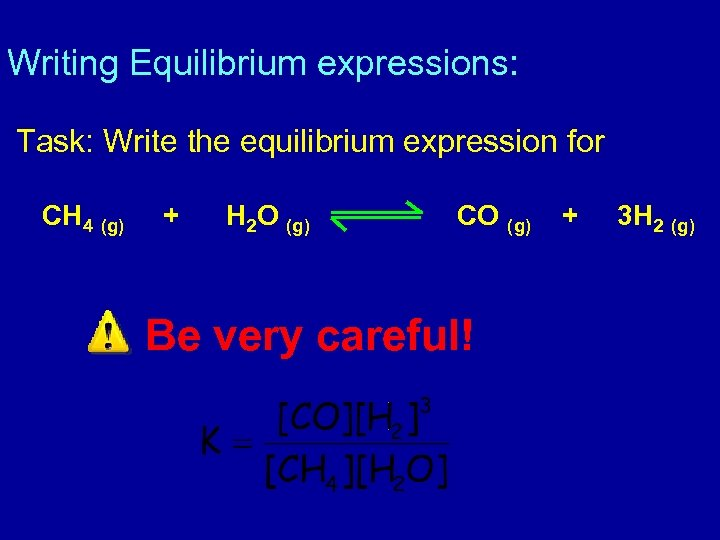Writing Equilibrium expressions: Task: Write the equilibrium expression for CH 4 (g) + H 2 O (g) CO (g) Be very careful! + 3 H 2 (g)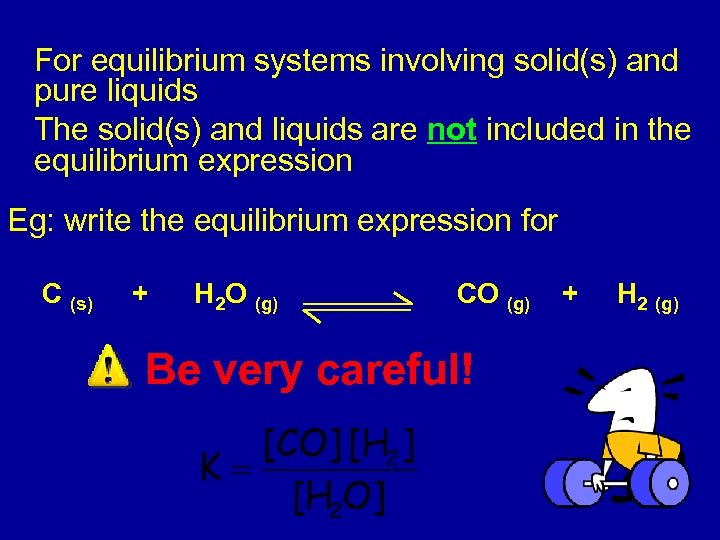For equilibrium systems involving solid(s) and pure liquids The solid(s) and liquids are not included in the equilibrium expression Eg: write the equilibrium expression for C (s) + H 2 O (g) CO (g) Be very careful! + H 2 (g)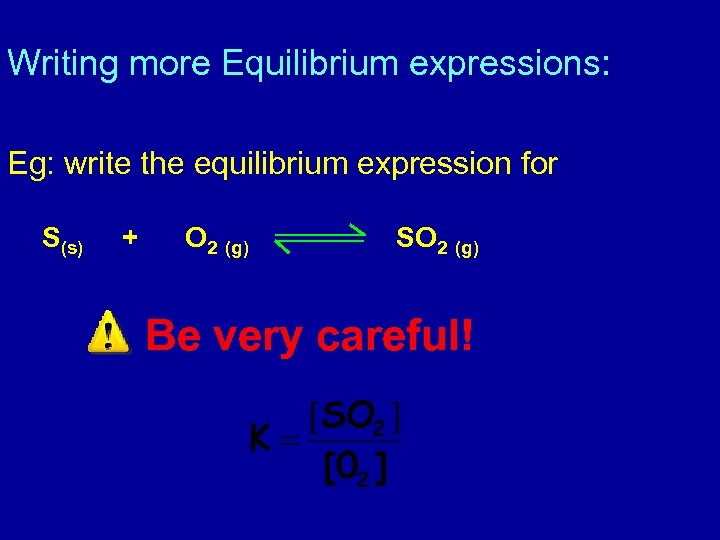Writing more Equilibrium expressions: Eg: write the equilibrium expression for S(s) + O 2 (g) SO 2 (g) Be very careful!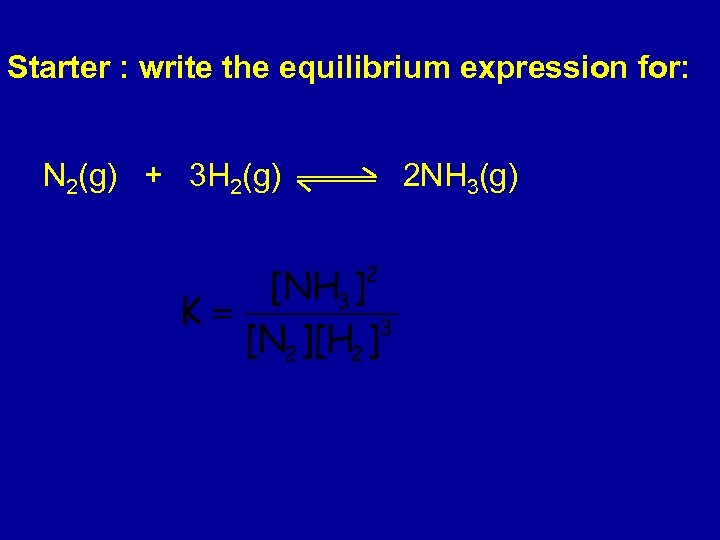Starter : write the equilibrium expression for: N 2(g) + 3 H 2(g) 2 NH 3(g)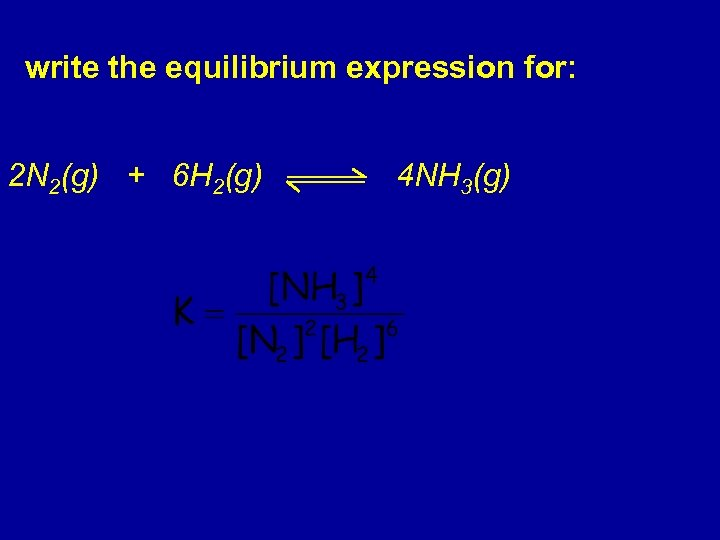write the equilibrium expression for: 2 N 2(g) + 6 H 2(g) 4 NH 3(g)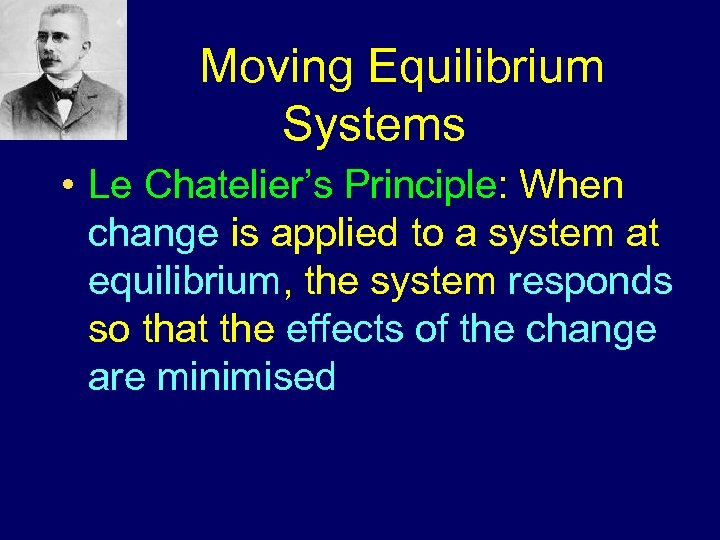Moving Equilibrium Systems • Le Chatelier’s Principle: When change is applied to a system at equilibrium, the system responds so that the effects of the change are minimised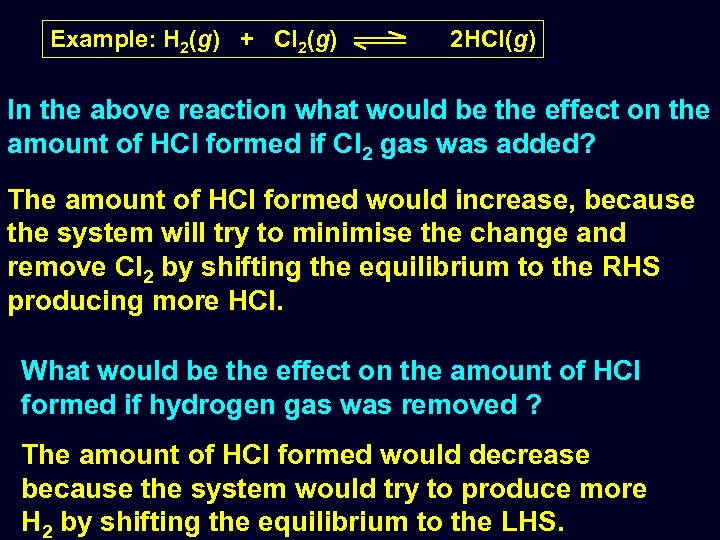Example: H 2(g) + Cl 2(g) 2 HCl(g) In the above reaction what would be the effect on the amount of HCl formed if Cl 2 gas was added? The amount of HCl formed would increase, because the system will try to minimise the change and remove Cl 2 by shifting the equilibrium to the RHS producing more HCl. What would be the effect on the amount of HCl formed if hydrogen gas was removed ? The amount of HCl formed would decrease because the system would try to produce more H 2 by shifting the equilibrium to the LHS.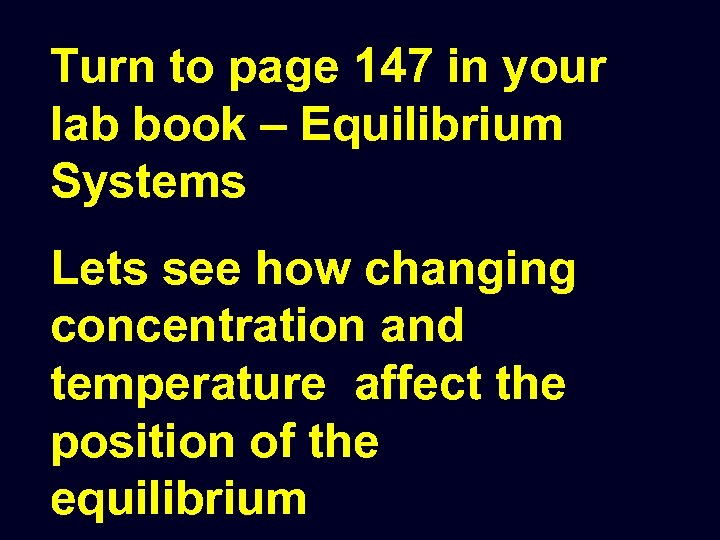Turn to page 147 in your lab book – Equilibrium Systems Lets see how changing concentration and temperature affect the position of the equilibrium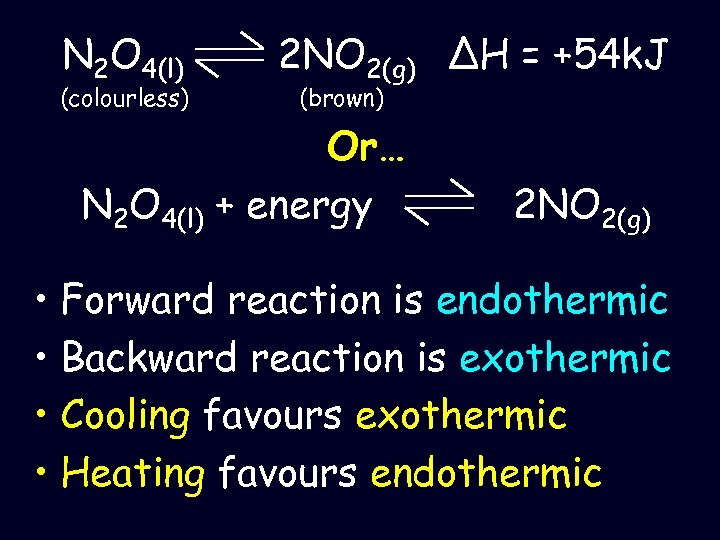N 2 O 4(l) (colourless) 2 NO 2(g) ΔH = +54 k. J (brown) Or… N 2 O 4(l) + energy 2 NO 2(g) • Forward reaction is endothermic • Backward reaction is exothermic • Cooling favours exothermic • Heating favours endothermic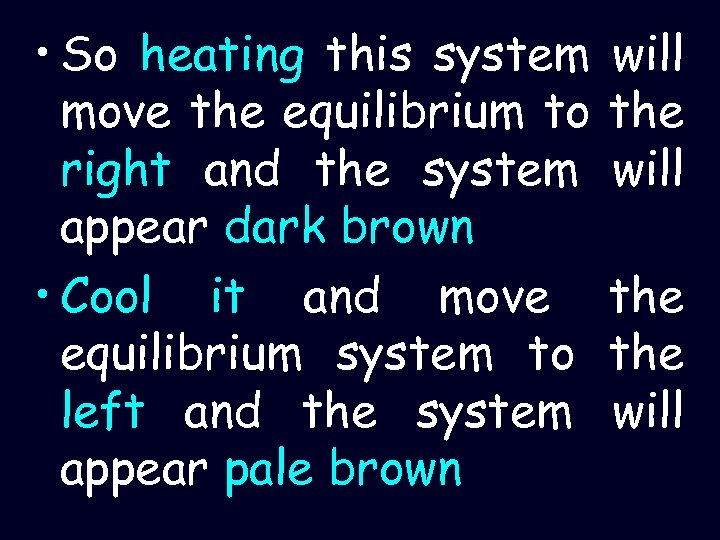• So heating this system move the equilibrium to right and the system appear dark brown • Cool it and move equilibrium system to left and the system appear pale brown will the the will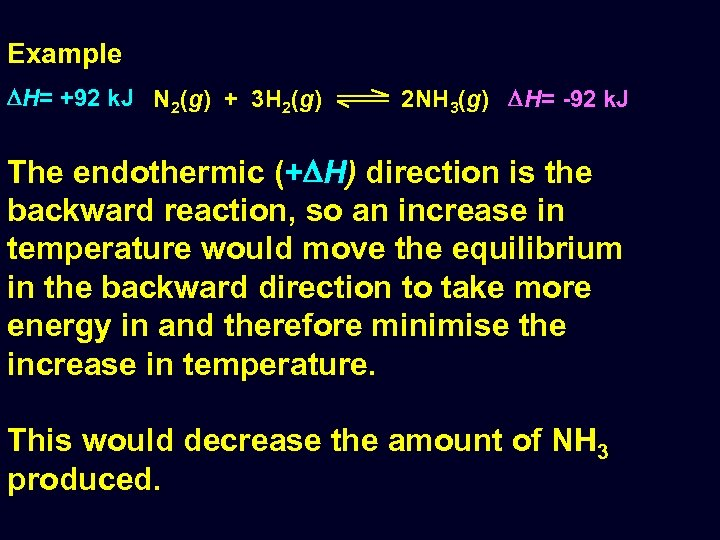Example H= +92 k. J N 2(g) + 3 H 2(g) 2 NH 3(g) H= -92 k. J The endothermic (+ H) direction is the backward reaction, so an increase in temperature would move the equilibrium in the backward direction to take more energy in and therefore minimise the increase in temperature. This would decrease the amount of NH 3 produced.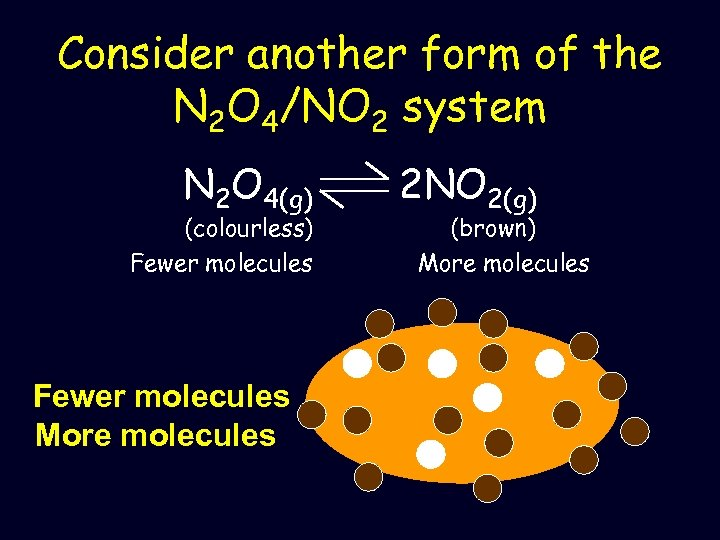Consider another form of the N 2 O 4/NO 2 system N 2 O 4(g) (colourless) Fewer molecules More molecules 2 NO 2(g) (brown) More molecules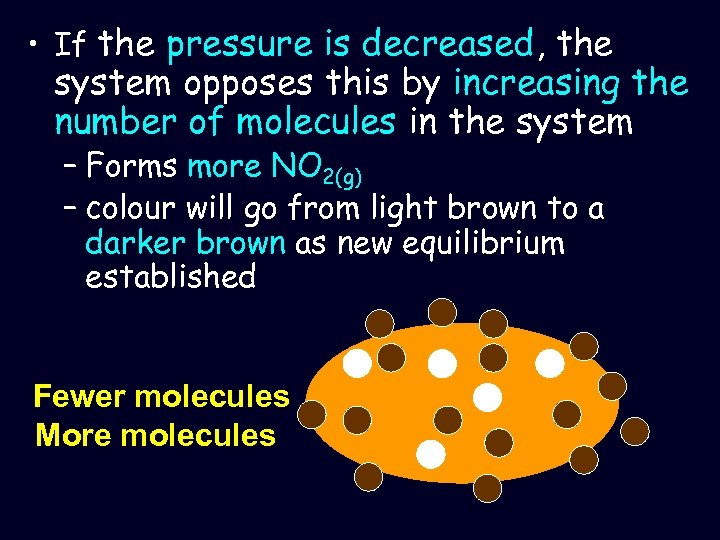• If the pressure is decreased, the system opposes this by increasing the number of molecules in the system – Forms more NO 2(g) – colour will go from light brown to a darker brown as new equilibrium established Fewer molecules More molecules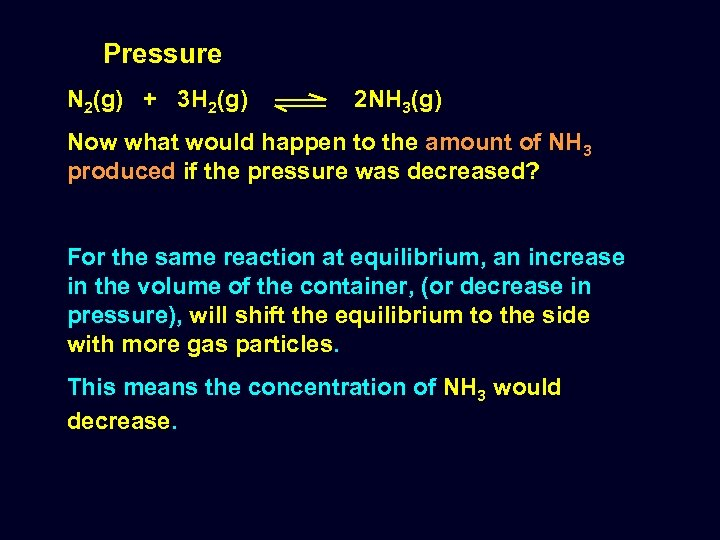Pressure N 2(g) + 3 H 2(g) 2 NH 3(g) Now what would happen to the amount of NH 3 produced if the pressure was decreased? For the same reaction at equilibrium, an increase in the volume of the container, (or decrease in pressure), will shift the equilibrium to the side with more gas particles. This means the concentration of NH 3 would decrease.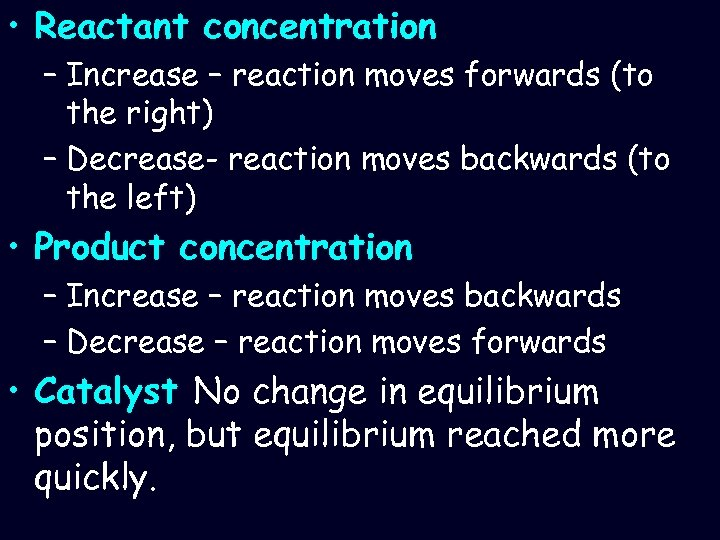• Reactant concentration – Increase – reaction moves forwards (to the right) – Decrease- reaction moves backwards (to the left) • Product concentration – Increase – reaction moves backwards – Decrease – reaction moves forwards • Catalyst No change in equilibrium position, but equilibrium reached more quickly.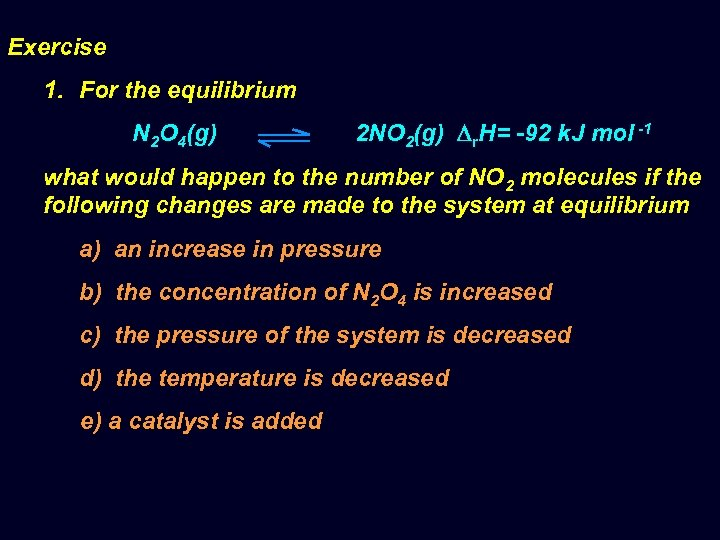Exercise 1. For the equilibrium N 2 O 4(g) 2 NO 2(g) r. H= -92 k. J mol -1 what would happen to the number of NO 2 molecules if the following changes are made to the system at equilibrium a) an increase in pressure b) the concentration of N 2 O 4 is increased c) the pressure of the system is decreased d) the temperature is decreased e) a catalyst is added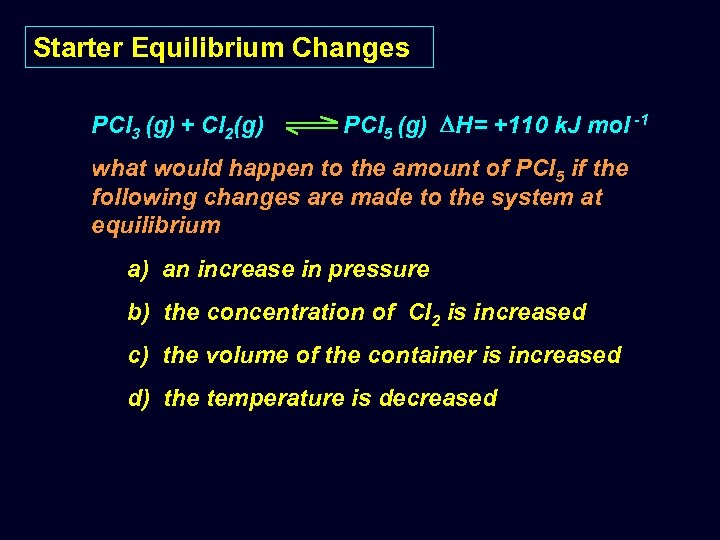Starter Equilibrium Changes PCl 3 (g) + Cl 2(g) PCl 5 (g) H= +110 k. J mol -1 what would happen to the amount of PCl 5 if the following changes are made to the system at equilibrium a) an increase in pressure b) the concentration of Cl 2 is increased c) the volume of the container is increased d) the temperature is decreased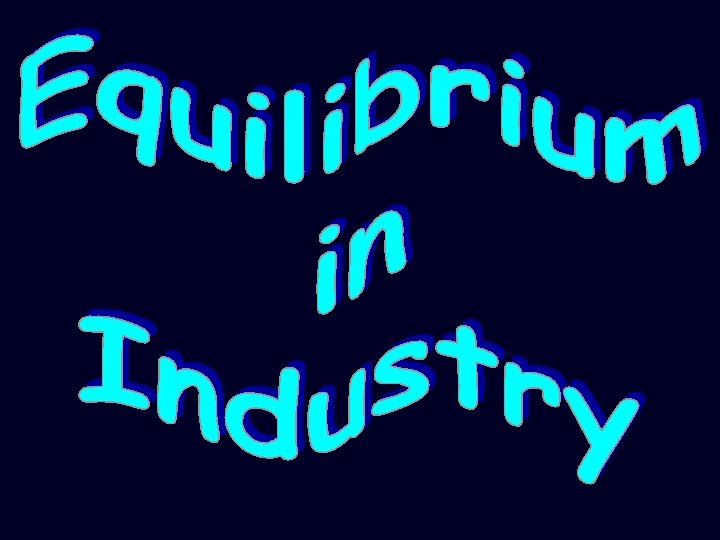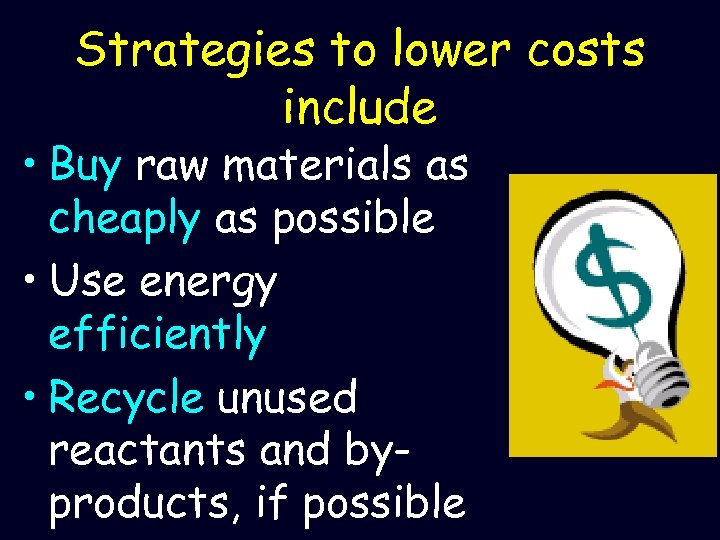Strategies to lower costs include • Buy raw materials as cheaply as possible • Use energy efficiently • Recycle unused reactants and byproducts, if possible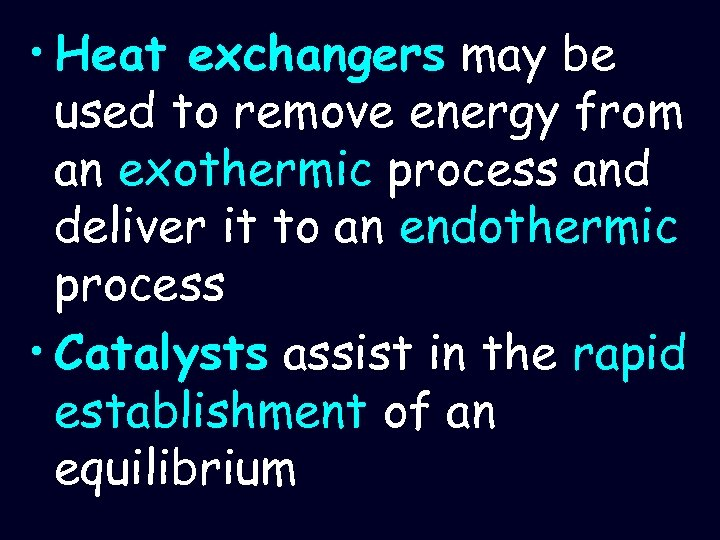• Heat exchangers may be used to remove energy from an exothermic process and deliver it to an endothermic process • Catalysts assist in the rapid establishment of an equilibrium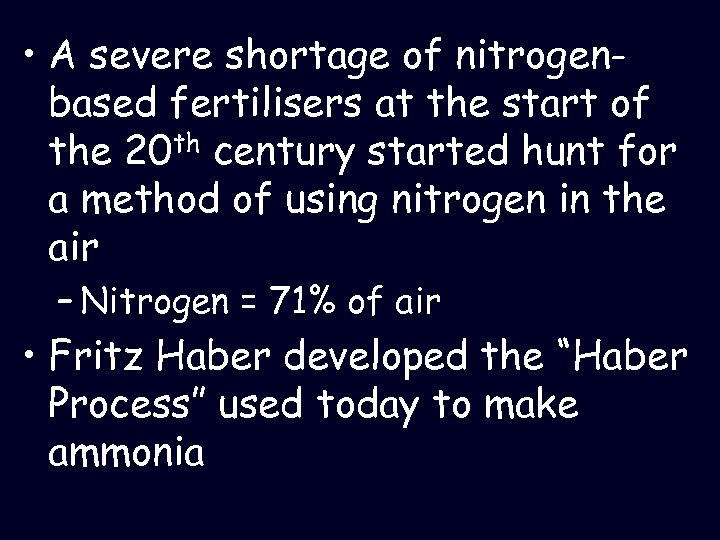• A severe shortage of nitrogenbased fertilisers at the start of the 20 th century started hunt for a method of using nitrogen in the air – Nitrogen = 71% of air • Fritz Haber developed the “Haber Process” used today to make ammonia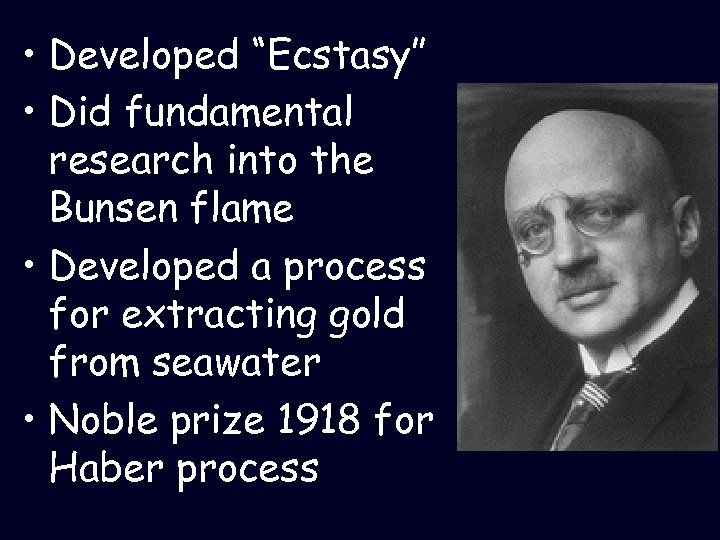• Developed “Ecstasy” • Did fundamental research into the Bunsen flame • Developed a process for extracting gold from seawater • Noble prize 1918 for Haber process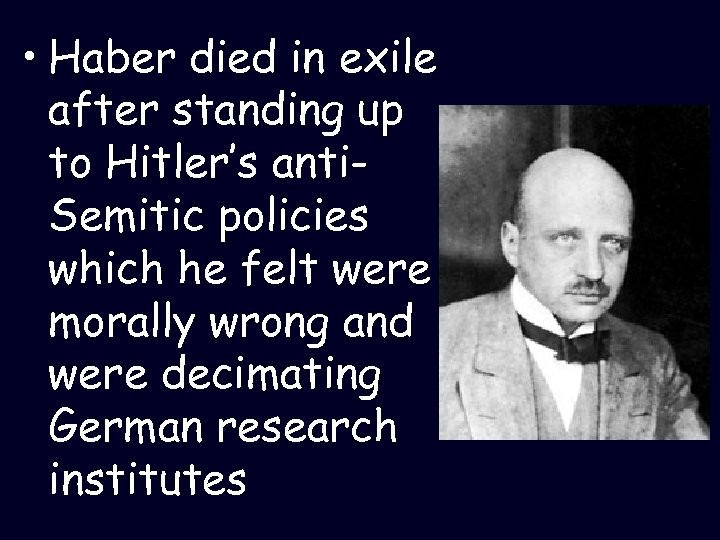• Haber died in exile after standing up to Hitler’s anti. Semitic policies which he felt were morally wrong and were decimating German research institutes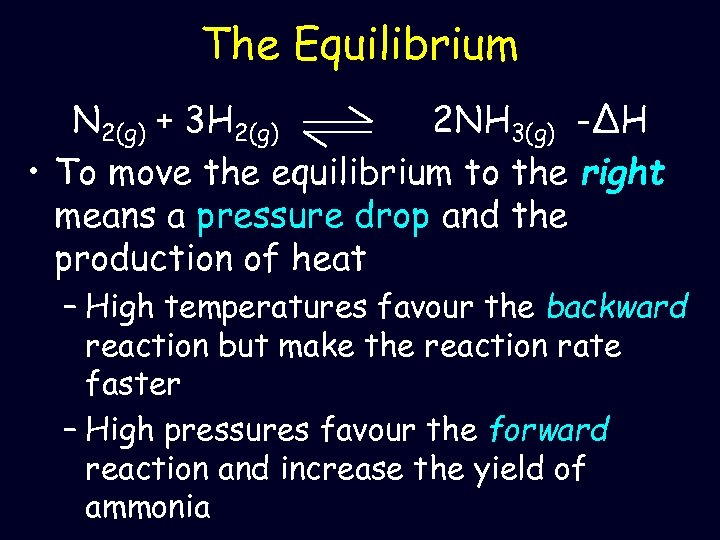The Equilibrium N 2(g) + 3 H 2(g) 2 NH 3(g) -ΔH • To move the equilibrium to the right means a pressure drop and the production of heat – High temperatures favour the backward reaction but make the reaction rate faster – High pressures favour the forward reaction and increase the yield of ammonia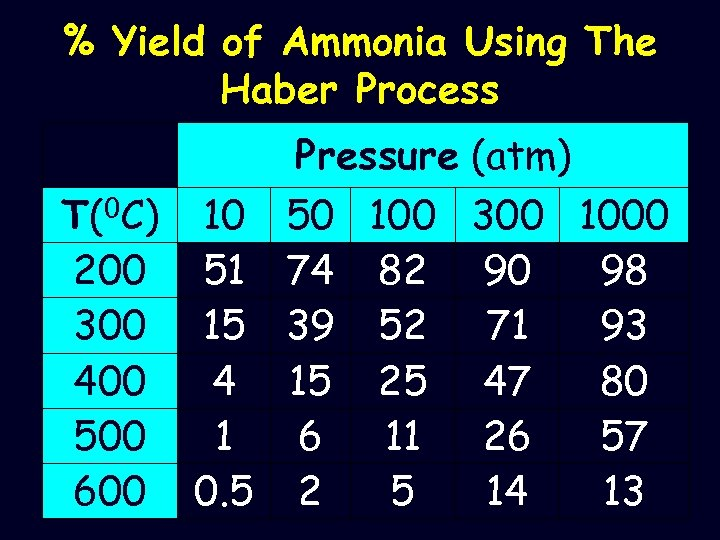% Yield of Ammonia Using The Haber Process Pressure (atm) T(0 C) 10 50 100 300 1000 200 51 74 82 90 98 300 15 39 52 71 93 400 4 15 25 47 80 500 1 6 11 26 57 600 0. 5 2 5 14 13